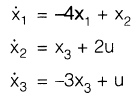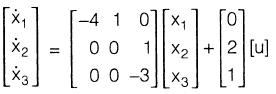Courses

# Control System - MCQ Test - 1

## 15 Questions MCQ Test GATE ECE (Electronics) 2022 Mock Test Series | Control System - MCQ Test - 1

Description
This mock test of Control System - MCQ Test - 1 for Electronics and Communication Engineering (ECE) helps you for every Electronics and Communication Engineering (ECE) entrance exam. This contains 15 Multiple Choice Questions for Electronics and Communication Engineering (ECE) Control System - MCQ Test - 1 (mcq) to study with solutions a complete question bank. The solved questions answers in this Control System - MCQ Test - 1 quiz give you a good mix of easy questions and tough questions. Electronics and Communication Engineering (ECE) students definitely take this Control System - MCQ Test - 1 exercise for a better result in the exam. You can find other Control System - MCQ Test - 1 extra questions, long questions & short questions for Electronics and Communication Engineering (ECE) on EduRev as well by searching above.
QUESTION: 1

### Determine C/R for the system given in figure below. Then put G3 = G1 G2 H2. Now the new transfer function will be: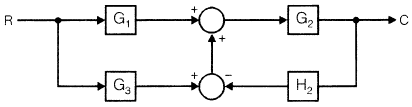Solution: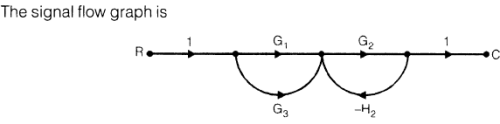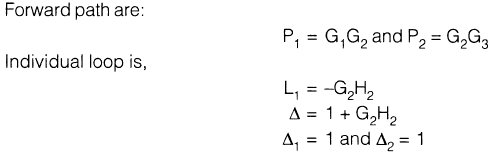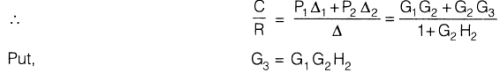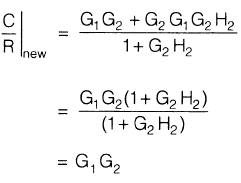QUESTION: 2

### The signal-flow graph shown in figure below has: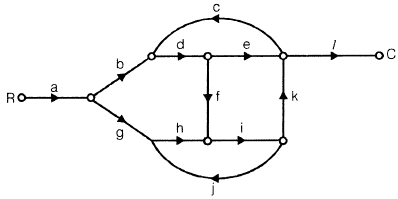Solution:

Forward paths are: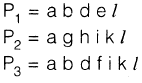Loops are: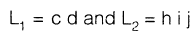*Answer can only contain numeric values
QUESTION: 3

### The unit impulse response of a system is h(t) = e-2t, t 0. For this system, the steady-state value of the output for unit step input is equal to

Solution: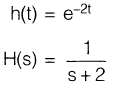and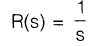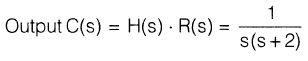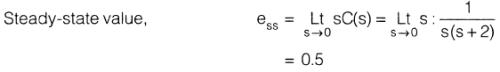QUESTION: 4

A control system has input r(t) and output c(t). If the input is first passed through a block

whose transfer function is a-2s and then applied to the system, the modified output will be

Solution: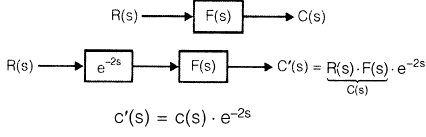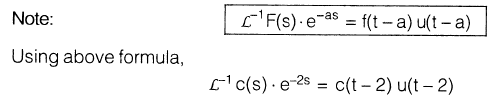QUESTION: 5

The open-loop transfer function of a feedback control system is given by G(s)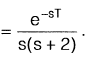Find the range of the values of T for stability.

Solution:

The characteristic equation of the system is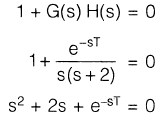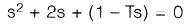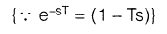s2 + s(2 — T) + 1 = 0

Routh's array becomes: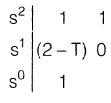The system will be stable if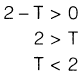QUESTION: 6

If the open-loop transfer function of a feedback system is given by

G(s) H(s) =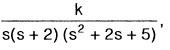, then the controid of the asymptotes will be

Solution:

Poles = 0, —2, —1 + 2j, —1 —2j

Total number of pole, P = 4

Total number of zero, Z = 0
∴           P — Z = 4

∴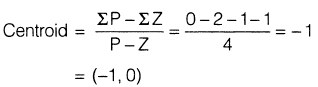QUESTION: 7

For the block diagram shown in figure below, the limiting value of k for stability of the inner loop is found to be X < k < Y. The overall system will be stable if and only if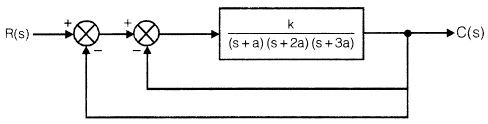Solution:

For inner loop: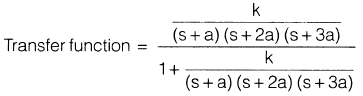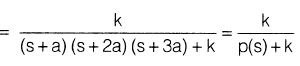let

For outer loop: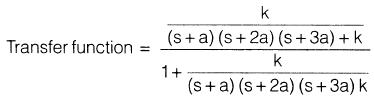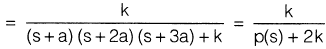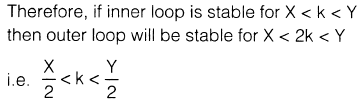QUESTION: 8

The closed loop transfer function of a control system has the following poles and zeros

Poles                                                       Zeros

P1 =    0.5                                                          Z1 = –7

P2 = –1.0                                                          Z2 = 9

P3 = –5

P4 = —10

The closed loop response can be closely approximated by considering which of the following?

Solution:

Because of concept of dominant pole. Here P1 and P2 are dominant pole and P3 and P4 are

insignificant poles.

QUESTION: 9

Match List-I (Type of compensator) with List-II (Polar plot) and select the correct answer using the code given below the lists:

List-I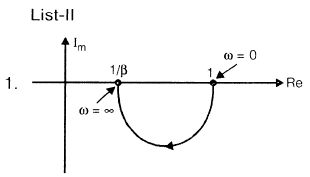1.Phase lead

2. Phase lag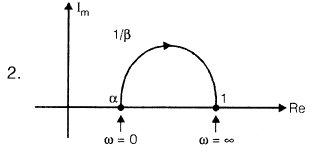3. Lead-lag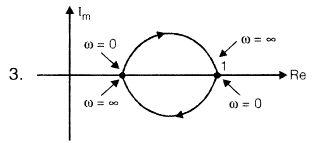A B C

(a)123

(b) 132

(c) 213

(d) 231

Solution:
QUESTION: 10

For the given network, the maximum phase lead 4m of Vo with respect to V1 is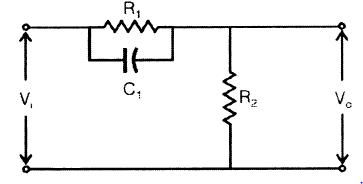Solution: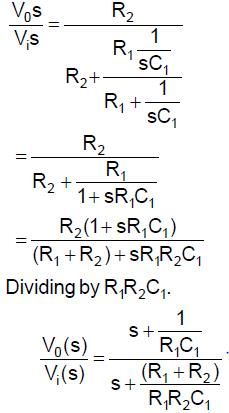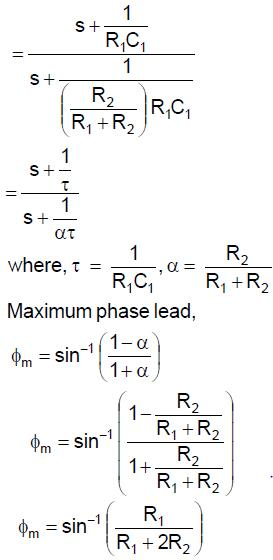QUESTION: 11

In the Bode-plot of a unity feedback control system, the value of phase of G(j@) at the gain

cross-over frequency is —125°. The phase margin of the system is

Solution:

φ at ωg C = —125°

Phase-margin (PM) = 180°+ φ

= 180°— 125°

= 55°

QUESTION: 12

The Nyquist plot for the function is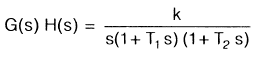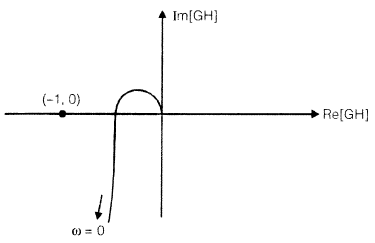The condition for stability is given by

Solution:
QUESTION: 13

The open-loop transfer function of unity feedback system is G(s) =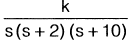. Thes (s + 2) (s + 10)range of k for which closed-loop system is stable.

Solution:

It is type-1 and order —3 system so the Nyquist plot is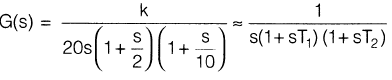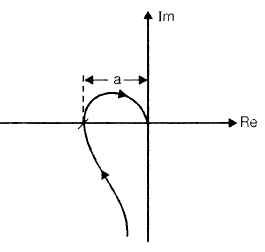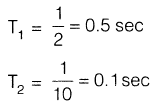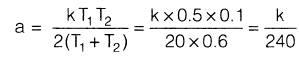For stability: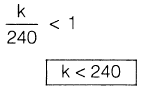QUESTION: 14

The Bode plot for minimum phase transfer function is:

Solution:

(a)

1. In option (a) Bode plot represents - Minimum phase transfer function
2. In option (b) Bode plot represents - Non-minimum phase transfer function
3. In option (c) Bode plot represents - All pass transfer function
QUESTION: 15

For the system shown below the state-space equation is X = A x + B u. The matrix A is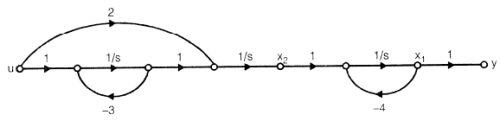Solution:

From the SFG